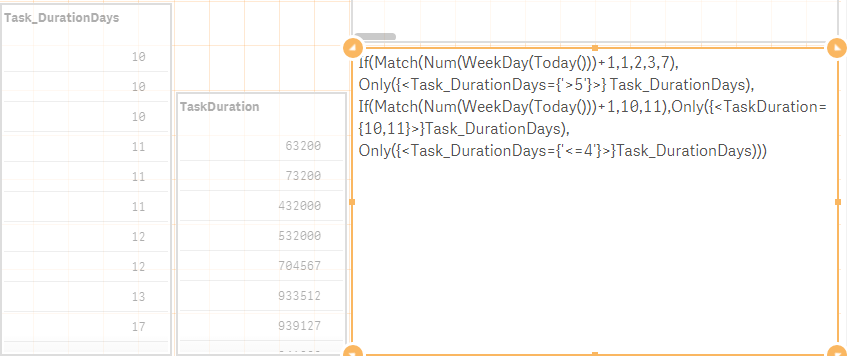New to Qlik Sense

Discussion board where members can get started with Qlik Sense.

Announcements
Valued Contributor III

current day logic

Hi all,

Antonio Mancini

I have a scenario like below  attached with sample data and with example.

and how can set the day of the week  ?  how to this in scripting?  and in  front end how to approach?

Thanks

Sonica

Tags (5)
1 Solution

Accepted Solutions
Honored Contributor III

Re: current day logic

Hi Sony,

if Today() =6 then DaysDuration >= 4  or <=4?

If(Match(Num(WeekDay(Today()))+1,1,2,3,7),Only({<DaysDuration={'>5'}>} DaysDuration),
If(Match(Num(WeekDay(Today()))+1,4,5),Only({<DaysDuration={4,5}>} DaysDuration),
Only({<DaysDuration={'<=4'}>} DaysDuration)))28 Replies
Contributor III

Re: current day logic

Hi,

Use the below expression..

=if(match(num(weekday(Today(1))),1,2,3,7), if([timeduration(in days)]>5, 'More Than 5 Days'),

if(match(num(weekday(Today(1))),4,5), if([timeduration(in days)]>3, 'More Than 3 Days'),

if(match(num(weekday(Today(1))),6), if([timeduration(in days)]=4, '4 Days or More'))

)

)

Honored Contributor III

Re: current day logic

Hi Sony,

if Today() =6 then DaysDuration >= 4  or <=4?

If(Match(Num(WeekDay(Today()))+1,1,2,3,7),Only({<DaysDuration={'>5'}>} DaysDuration),
If(Match(Num(WeekDay(Today()))+1,4,5),Only({<DaysDuration={4,5}>} DaysDuration),
Only({<DaysDuration={'<=4'}>} DaysDuration)))Valued Contributor III

Re: current day logic

thanks for quick response  prashanth and Antonio.

Give me some time I  will check and come back to this logic

Contributor III

Re: current day logic

Also attached the sample app with your data

Valued Contributor III

Re: current day logic

thanks but If I want to create it backend how to create?

Valued Contributor III

Re: current day logic

hi Sir,

if Today() =6 then DaysDuration >= 4  or <=4?

logic :for this ----> if day is 6   and duration  =4 and >4

and how to set the weekday in script?  how to use variable to set that?

Highlighted
Honored Contributor III

Re: current day logic

In Script

LET vDay = Num(WeekDay(Today()))+1;

Expression :

If(Match(vDay,1,2,3,7),Only({<DaysDuration={'>5'}>} DaysDuration),
If(Match(vDay,4,5),Only({<DaysDuration={4,5}>} DaysDuration),
Only({<DaysDuration={'>=4'}>} DaysDuration)))

Valued Contributor III

Re: current day logicin my data it is not working sir,

in my data duration column coming as seconds so I  converted like below

the above both expressions are not giving any result